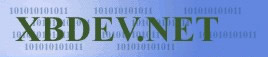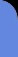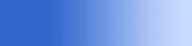Saturday July 4, 2020
 home | about | contact | DonationsFractals and the Mandelbulb Kenwright

Fractal make you see everything differently. Not only are fractals incredibly powerful, but they are also beautiful and fun. In particular, graphical fractals possess infinite detail combined with unpredictability, that is really amazing. Once you get past all of the mathematics and visualization challenges, you'll never look at the world the same again ;)

A particular fractal with interesting visual propoperties is the Mandelbulb.

## Famous 2D Mandelbrot Equation

2D Mandelbrot equation:

\$z{n+1} = z{n}^2+c\$.

Squaring complex numbers has a simple geometric interpretation: if the complex number is represented in polar coordinates, squaring the number corresponds to squaring the length, and doubling the angle (to the real axis).

Taking the Mandelbrot equation to higher dimensions leads us to what is now known as the Mandelbulb fractal.

```# 2D Cross-section Of (3D) Mandelbrot Fractal import math import random from PIL import Image imgx = 256 # 512 imgy = 256 # 512 image = Image.new("RGB", (imgx, imgy)) pixels = image.load() n = 8 # drawing area (xa < xb & ya < yb) xa = -1.5 xb = 1.5 ya = -1.5 yb = 1.5 maxIt = 256 # 256 # max number of iterations allowed pi2 = math.pi * 2.0 # random rotation angles to convert 2d plane to 3d plane xy = 0.2 * pi2; # random.random() * pi2 xz = 0.2 * pi2; # random.random() * pi2 yz = 0.2 * pi2; # random.random() * pi2 sxy = math.sin(xy) ; cxy = math.cos(xy) sxz = math.sin(xz) ; cxz = math.cos(xz) syz = math.sin(yz) ; cyz = math.cos(yz) origx = (xa + xb) / 2.0 ; origy = (ya + yb) / 2.0 for ky in range(imgy): b = ky * (yb - ya) / (imgy - 1) + ya for kx in range(imgx): a = kx * (xb - xa) / (imgx - 1) + xa x = a ; y = b ; z = 0.5 # 3d rotation around center of the plane x = x - origx ; y = y - origy x0=x*cxy-y*sxy;y=x*sxy+y*cxy;x=x0 # xy-plane rotation x0=x*cxz-z*sxz;z=x*sxz+z*cxz;x=x0 # xz-plane rotation y0=y*cyz-z*syz;z=y*syz+z*cyz;y=y0 # yz-plane rotation x = x + origx ; y = y + origy cx = x ; cy = y ; cz = z for i in range(maxIt): r = math.sqrt(x * x + y * y + z * z) t = math.atan2(math.hypot(x, y), z) p = math.atan2(y, x) rn = r ** n x = rn * math.sin(t * n) * math.cos(p * n) + cx y = rn * math.sin(t * n) * math.sin(p * n) + cy z = rn * math.cos(t * n) + cz if x * x + y * y + z * z > 150.0: break if i > 1 and i <= 50: ss = 128 + int( 128.0 * (i/50.0) ) pixels[kx, ky] = (ss, 0, 0) if i > 50 and i <= 100: ss = 128 + int( 128.0 * ((i-100)/50.0) ) pixels[kx, ky] = (0, ss, 0) if i > 100 and i <= 150: ss = 128 + int( 128.0 * ((i-100)/100.0) ) pixels[kx, ky] = (0, 0, ss) if i == 2: pixels[kx, ky] = (255, 255, 255 ) image.save("Mandelbulb.png", "PNG") ```

Output for the above Python program. Show the cross sectional view of a Mandelbulb.The various depths are shown in different colours.

# MandelBulb Ray Tracer (Stripped)

A minimum working Mandelbulb ray tracing implementation. All you need is a C++ compiler. Of course, you could port it to Python, as it's relatively straightforward, but it would be slow! It's slow in C++, it takes a few seconds to create the image in C++, so it might take a few minutes in Python.

The implementation should hopefully let you see how it all fits together. Also a fun demo for you to tinker with and experiment with as you learn about geometric fractals.

The following implementation creates a ppm image file. You should be able to open this image file in most image editors (e.g., XNView is a free image viewer/formatter if you don't have Photoshop).``````

/*
Minimal working

PPM image format is a simple and uncomplicated way to generate an image without
requiring any external libraries.  Easy to open and convert to another format
(e.g., open and save as jpg using xnview).

*/

#define _CRT_SECURE_NO_WARNINGS

// constants to tweak and control the fractal output
#define BAILOUT        100
#define EPS            0.001
#define mmaxIterations 10

#define maxDetailIter  100
// e.g., try and change this value to 5 instead
// and see what the generated output looks like
#define myPower        12

#include
#include
#include
#include

using namespace std;

#define M_PI 3.14159265358979323846264338327950288

inline double clamp(double x) { return x < 0 ? 0 : x>1 ? 1 : x; }
inline double min(double x, double y){ if (xy) return x; return y; }
inline int toInt(double x) { return int(pow(clamp(x), 1 / 2.2) * 255 + .5); }
inline double clamp(const double ff, double lo, double hi)
{
if (ff > hi) return hi;
if (ff < lo) return lo;
return ff;
}

struct vec3 {
double x, y, z;                  // position, also color (r,g,b)
vec3(double x_ = 0, double y_ = 0, double z_ = 0) { x = x_; y = y_; z = z_; }
vec3 operator+(const vec3 &b) const { return vec3(x + b.x, y + b.y, z + b.z); }
vec3 operator-(const vec3 &b) const { return vec3(x - b.x, y - b.y, z - b.z); }
vec3 operator*(double b) const { return vec3(x*b, y*b, z*b); }
vec3 mult(const vec3 &b) const { return vec3(x*b.x, y*b.y, z*b.z); }
vec3& norm() { return *this = *this * (1 / sqrt(x*x + y*y + z*z)); }
double dot( const vec3 &b) const { return x*b.x + y*b.y + z*b.z; }
vec3 cross (vec3&b) { return vec3(y*b.z - z*b.y, z*b.x - x*b.z, x*b.y - y*b.x); }
};

struct vec4 {
double x, y, z, w;
vec4(const vec3& v, double _w){ x=v.x, y=v.y, z=v.z, w=_w; }
vec4(double x_ = 0, double y_ = 0, double z_ = 0, double w_ = 0) { x = x_; y = y_; z = z_; w = w_; }
vec4 operator+(const vec4 &b) const { return vec4(x + b.x, y + b.y, z + b.z, w + b.w); }
vec4 operator-(const vec4 &b) const { return vec4(x - b.x, y - b.y, z - b.z, w - b.w); }
vec4 operator*(double b) const { return vec4(x*b, y*b, z*b, w*b); }
vec4 mult(const vec4 &b) const { return vec4(x*b.x, y*b.y, z*b.z, w*b.w); }
vec4& norm() { return *this = *this * (1 / sqrt(x*x + y*y + z*z + w*w)); }
double dot(const vec4 &b) const { return x*b.x + y*b.y + z*b.z, w*b.w; }

};

double dot(const vec3&a, const vec3 &b) { return a.dot(b); }
double length(const vec3& v) { return sqrt(v.dot(v)); }
double length(const vec4& v) { return sqrt(v.dot(v)); }
vec3 normalize(const vec3& v) {
double len = length(v);
return vec3( v.x/len, v.y/len, v.z/len );
}

/*
These two functions, MainBulb(..) and IntersectMBulb(..)
do all of the work.  Responsible for the fractal shape/details
*/

vec4 MainBulb(vec4 v)
{
double r = length( vec3(v.x, v.y, v.z) );
if (r>BAILOUT) return v;
double theta = acos(clamp(v.z / r, -1.0, 1.0))*myPower;
double phi = atan2(v.y, v.x)*myPower;
v.w = pow(r, myPower - 1.0)*myPower*v.w + 1.0;

double zr = pow(r, myPower);

vec3 vv = vec3(sin(theta)*cos(phi), sin(phi)*sin(theta), cos(theta))*zr;
return vec4(vv.x, vv.y, vv.z, v.w);
}

double IntersectMBulb(vec3& rO, vec3& rD, vec4& trap)
{
double dist;
for (int i = 0; iBAILOUT) break;

v = MainBulb(v) + vec4(rO.x, rO.y, rO.z, 0.0);

va = vec3(v.x, v.y, v.z);
vec4 v4 (abs(va.x), abs(va.y), abs(va.z), dot(va, va));
trap.x = min(trap.x, v4.x);
trap.y = min(trap.y, v4.y);
trap.z = min(trap.z, v4.z);
trap.w = min(trap.w, v4.w);
}

dist = 0.5*log(r)*r / v.w;
rO = rO + rD * dist;
if (dist < EPS) break;
}
return dist;
}

/*
Hacky code to work out an approximate normal for our
geometry (without lighting it looks horrible)
*/
vec3 NormEstimateMB(vec3 p) {

double ddd = 0.000001;

vec4 g0 = vec4(p, .0);
vec4 gx = vec4(p + vec3(ddd, 0, 0), .0);
vec4 gy = vec4(p + vec3(0, ddd, 0), .0);
vec4 gz = vec4(p + vec3(0, 0, ddd), .0);

vec4 v0 = g0;
vec4 vx = gx;
vec4 vy = gy;
vec4 vz = gz;

double ln;
for (int i = 0; i < 100; i++) {
g0 = MainBulb(g0) + v0;
gx = MainBulb(gx) + vx;
gy = MainBulb(gy) + vy;
gz = MainBulb(gz) + vz;
ln = length( vec3(g0.x, g0.y, g0.z) );
if (ln>BAILOUT) break;
}

//float ln    = length( vec3(g0.x, g0.y, g0.z) );
double gradX = length( vec3(gx.x, gx.y, gx.z) ) - ln;
double gradY = length( vec3(gy.x, gy.y, gy.z) ) - ln;
double gradZ = length( vec3(gz.x, gz.y, gz.z) ) - ln;
//N = normalize(vec3(length(gx-g0), length(gy-g0), length(gz-g0)));
}

/*
Good old Phong lighting function
*/
vec3 Phong(vec3 light, vec3 eye, vec3 pt, vec3 N)
{
vec3 diffuse = vec3(0.40, 0.95, 0.25); // base color of shading
const int specularExponent = 10;       // shininess of shading
const double specularity = 0.45;       // amplitude of specular highlight

vec3 L = normalize(light - pt);        // find the vector to the light
vec3 E = normalize(eye - pt);          // find the vector to the eye
double  NdotL = dot(N, L);             // find the cosine of the angle between light and normal
vec3 R = L - N * 2 * NdotL;            // find the reflected vector

diffuse = diffuse + N*0.1;             // add some of the normal for 'effect'

return diffuse * max(NdotL, 0.0) + specularity*pow(max(dot(E, R), 0), specularExponent);
}

/*
Program entry point - loop over all the image pixels
and work out the color (i.e., based on a ray we shoot
at the Mandelbulb fractal/intersection).
*/
int main(int argc, char *argv[]) {

int w = 1024, h = 1014; // # default resolution
if ( argc == 2 ) w = h = atoi(argv); // # resolution

vec3 *c = new vec3[w*h]; // output colors

#pragma omp parallel for schedule(dynamic, 1) private(r)       // OpenMP
for (int y = 0; y```

 [Wikipedia Mandelbulb](https://en.wikipedia.org/wiki/Mandelbulb) - Explains the history and the mathematics (helps you understand where and why of the equations in the code)
 [WebGL MandelBulb Ray Marching 3D rendering](https://github.com/BrutPitt/wglMandelBulber) [Nice web-based demo on GitHub]
 Dual-Quaternion Julia Fractals (Kenwright) - Exploring fractals (alternative mathematical models and methods for representing and defining geometric surfaces)
 [Python Mandelbulb Slice Receipe](http://code.activestate.com/recipes/578198-mandelbulb-fractal/) Sweet little python program to show a minimal working example of a mandelbulb 'slice'
 [Daniel White's site](https://www.skytopia.com/project/fractal/mandelbulb.html) Mandelbulb and its history, very well documented details, and continue to discuss various distance estimators for the concept

```
 Visitor: 9534626  { 209.237.238.175 } Copyright (c) 2002-2020 xbdev.net - All rights reserved. Designated tutorial and software are the property of their respective owners.